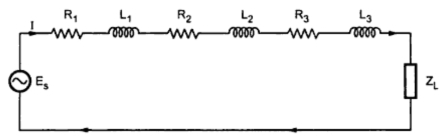### Transmission Line Constants

Transmission lines are the circuits with the distributed constants such as resistance, inductance, conductance and capacitance distributed along the length of transmission line. These constant concentrated at any point. Their values are normally expressed as per kilometer length of line.
Consider the distributed circuit shown in the Fig. 1. The analysis of this circuit can be made by lumping the parameters which is shown in the Fig. 2.Fig. 1Fig. 2
The analysis of circuit becomes easier by lumping the parameters as the same current is passing through each element of resistance and inductance.
Similarly the circuits shown in the Fig. 3(a) and (b) are seen to be equivalent as the same voltage is applied across each conductance and capacitance.Fig. 3
But if the elements are distributed as shown in the Fig. 4. then the elements can not be lumped as the same current is not flowing through series element and the voltage across different element changes from one section to other.
Consider the equivalent circuit of a 2-wire transmission line having resistance, inductance, capacitance and conductance associated with it as shown in the Fig. 4.Fig. 4
The line is divided into large number of small sections of unit length. The upper and lower part of each section carries same current. Hence we have the equivalent circuit as shown in the Fig. 5.
Here                   R1T =  R1 +R1',  R2T = R2 + R2'
Similarly              L1T = L1 +L1'   , L2T = L2 + L2' etcFig. 5
The resistance per unit length of the line is say R where R1T = R2T = R3T = R4T ... = R, ohm/metre. We have L1T = L1T = L1T = ... = L, henry/metre
G = G = ... G, mho/metre
C = C2 = .... C, farad/metre
In case of 2 wire transmission system the line parameters are expressed on loop basis while in case of 3 phase transmission system the parameters are represented on per conductor basis.
The physical length of the transmission line does not indicate that the line is short or long but it is based on comparison with the wavelength (λ) of the source.